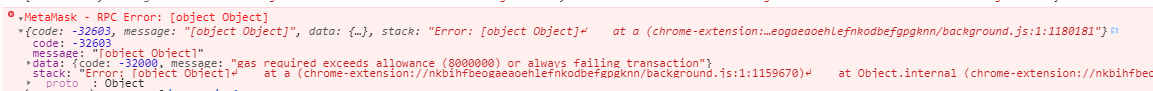### 优化 DeFi 合约利息计算 gas 消耗## 常规计算利息方法

\$yi = B * yr \$

\$ YI = yi + B = B *( 1 + yr) \$

\$ YI = B *( 1 + dr)^{365} \$

\$ DI_t = B * dR^n \$

\$ DI_{100} = B * dR^{100} \$

\$dR_i\$ 表示某一时间段利率, \$n_i\$ 表示\$dR_i\$持续的时间, \$t_i\$ 表示某段结束时刻，即：\$tn = n1+n2+n3+...+ni\$

\$ DI_{t1} = B * dR_1^{n1} \$

\$ DI{t2} = DI{t1} * dR_2^{n2} \$

\$ DI{t3} = DI{t2} * dR_3^{n3} \$

## 使用单位累积利率

\$ DI_{t1} = B * \$ ( \$dR_1^{n1}\$ )

\$ DI_{t2} = B \$ ( \$dR_1^{n1} dR_2^{n2}\$ )

\$ DI_{t3} = B \$ ( \$dR_1^{n1} dR_2^{n2} * dR_3^{n3} \$)

\$ DI = B * chi \$

\$ chi{ti} = chi{n-1} * dR ^{ni} \$

• 发表于 2020-03-14 18:03
• 阅读 ( 2512 )
• 学分 ( 242 )
• 分类：智能合约